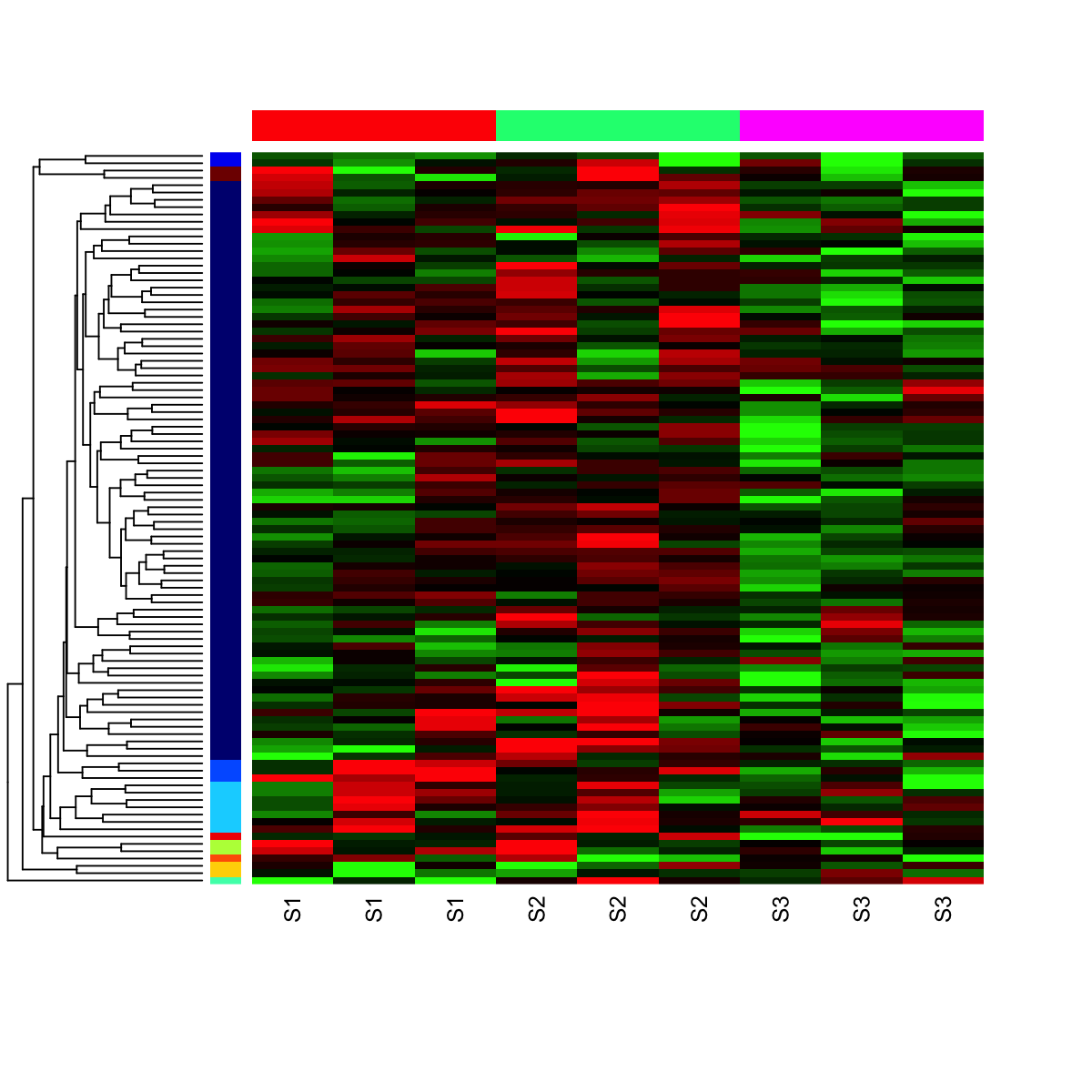## Function to visualise input data matrix using heatmap

### Description

`visHeatmap` is supposed to visualise input data matrix using heatmap. Note: this heatmap displays matrix in a bottom-to-top direction

### Usage

```visHeatmap(data, scale = c("none", "row", "column"), row.metric = c("none", "pearson",
"spearman", "kendall", "euclidean", "manhattan", "cos", "mi"), row.method = c("ward",
"single", "complete", "average", "mcquitty", "median", "centroid"), column.metric = c("none",
"pearson", "spearman", "kendall", "euclidean", "manhattan", "cos", "mi"), column.method = c("ward",
"single", "complete", "average", "mcquitty", "median", "centroid"), colormap = c("bwr",
"jet", "gbr", "wyr", "br", "yr", "rainbow", "wb"), ncolors = 64, zlim = NULL,
row.cutree = NULL, row.colormap = c("rainbow"), column.cutree = NULL, column.colormap = c("rainbow"),
...)```

### Arguments

data
an input gene-sample data matrix used for heatmap
scale
a character indicating when the input matrix should be centered and scaled. It can be one of "none" (no scaling), "row" (being scaled in the row direction), "column" (being scaled in the column direction)
row.metric
distance metric used to calculate the distance metric between rows. It can be one of "none" (i.e. no dendrogram between rows), "pearson", "spearman", "kendall", "euclidean", "manhattan", "cos" and "mi". See details at http://suprahex.r-forge.r-project.org/sDistance.html
row.method
the agglomeration method used to cluster rows. This should be one of "ward", "single", "complete", "average", "mcquitty", "median" or "centroid". See 'Note' below for details
column.metric
distance metric used to calculate the distance metric between columns. It can be one of "none" (i.e. no dendrogram between rows), "pearson", "spearman", "kendall", "euclidean", "manhattan", "cos" and "mi". See details at http://suprahex.r-forge.r-project.org/sDistance.html
column.method
the agglomeration method used to cluster columns. This should be one of "ward", "single", "complete", "average", "mcquitty", "median" or "centroid". See 'Note' below for details
colormap
short name for the colormap. It can be one of "jet" (jet colormap), "bwr" (blue-white-red colormap), "gbr" (green-black-red colormap), "wyr" (white-yellow-red colormap), "br" (black-red colormap), "yr" (yellow-red colormap), "wb" (white-black colormap), and "rainbow" (rainbow colormap, that is, red-yellow-green-cyan-blue-magenta). Alternatively, any hyphen-separated HTML color names, e.g. "blue-black-yellow", "royalblue-white-sandybrown", "darkgreen-white-darkviolet". A list of standard color names can be found in http://html-color-codes.info/color-names
ncolors
the number of colors specified over the colormap
zlim
the minimum and maximum z/patttern values for which colors should be plotted, defaulting to the range of the finite values of z. Each of the given colors will be used to color an equispaced interval of this range. The midpoints of the intervals cover the range, so that values just outside the range will be plotted
row.cutree
an integer scalar specifying the desired number of groups being cut from the row dendrogram. Note, this optional is only enabled when the row dengrogram is built
row.colormap
short name for the colormap to color-code the row groups (i.e. sidebar colors used to annotate the rows)
column.cutree
an integer scalar specifying the desired number of groups being cut from the column dendrogram. Note, this optional is only enabled when the column dengrogram is built
column.colormap
short name for the colormap to color-code the column groups (i.e. sidebar colors used to annotate the columns)
...
additional graphic parameters. Type ?heatmap for the complete list.

invisible

### Note

The clustering methods are provided:

• "ward": Ward's minimum variance method aims at finding compact, spherical clusters
• "single": The single linkage method (which is closely related to the minimal spanning tree) adopts a 'friends of friends' clustering strategy
• "complete": The complete linkage method finds similar clusters
• "average","mcquitty","median","centroid": These methods can be regarded as aiming for clusters with characteristics somewhere between the single and complete link methods. Two methods "median" and "centroid" are not leading to a monotone distance measure, or equivalently the resulting dendrograms can have so called inversions (which are hard to interpret)

### Examples

```# 1) generate data with an iid matrix of 100 x 9
data <- cbind(matrix(rnorm(100*3,mean=0,sd=1), nrow=100, ncol=3),
matrix(rnorm(100*3,mean=0.5,sd=1), nrow=100, ncol=3),
matrix(rnorm(100*3,mean=-0.5,sd=1), nrow=100, ncol=3))
colnames(data) <- c("S1","S1","S1","S2","S2","S2","S3","S3","S3")

# 2) prepare colors for the column sidebar
lvs <- unique(colnames(data))
lvs_color <- visColormap(colormap="rainbow")(length(lvs))
my_ColSideColors <- sapply(colnames(data), function(x)
lvs_color[x==lvs])

# 3) heatmap with row dendrogram (with 10 color-coded groups)
visHeatmap(data, row.metric="euclidean", row.method="average",
colormap="gbr", zlim=c(-2,2),
ColSideColors=my_ColSideColors, row.cutree=10, row.colormap="jet",
labRow=NA)```

## Source code

`visHeatmap.r`

## Source man

`visHeatmap.Rd` `visHeatmap.pdf`

`visHeatmap`